## LetsPlayMaths.Com

WELCOME TO THE WORLD OF MATHEMATICS

# Class 2 Multiplication

Basics of Multiplication

Multiplication Tables

Multiplication of 2-Digit Number by 1-Digit Number

Multiplication of 3-Digit Number by 1-Digit Number

Multiplication by 10,20,30

Word Problem

Multiplication Test

Multiplication Worksheet

## Basics of Multiplication

In class-I, we have learnt some basic concept of multiplication. Here we will go little in depth of multiplication.As we know, multiplication is nothing, but the repeated addition and it denoted as ‘X’ sign. The result of multiplication is known as product.We have 3 balls in 3 groups.
So total number of balls = 3 + 3 + 3 = 9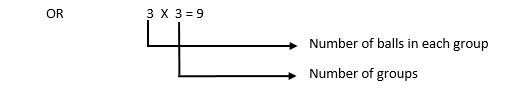## Multiplication Tables

In class-I, we have learnt multiplication table up to 5. Here, we will learn till 15. Try to memorize all the
below mentioned tables for quicker problem resolution.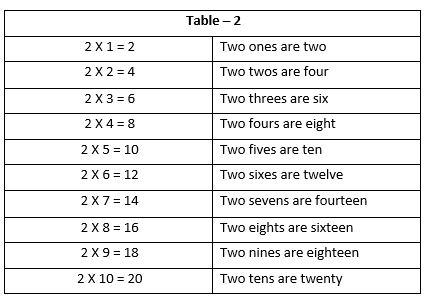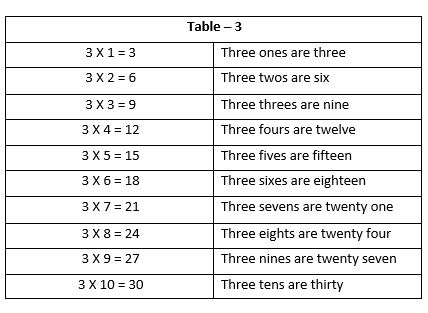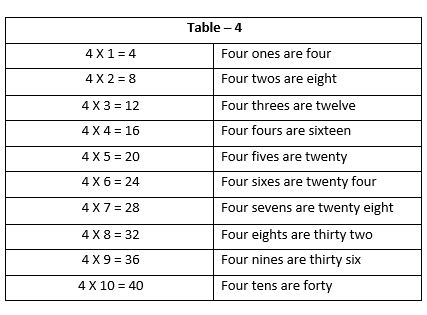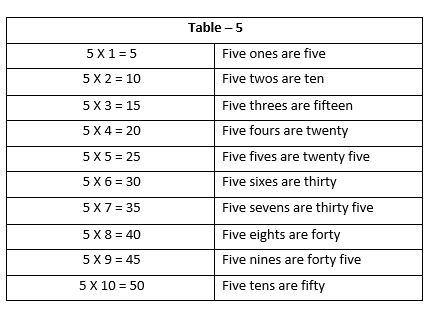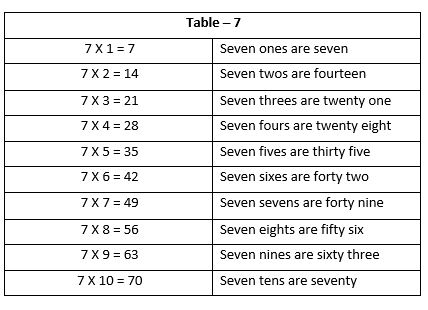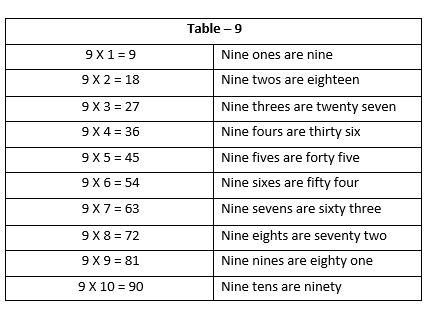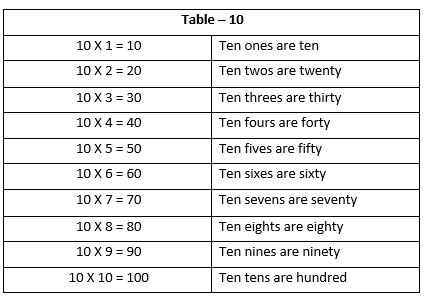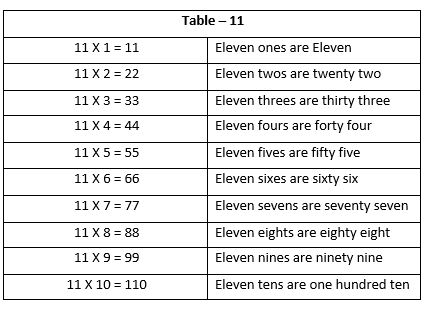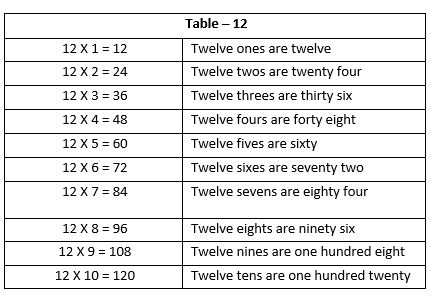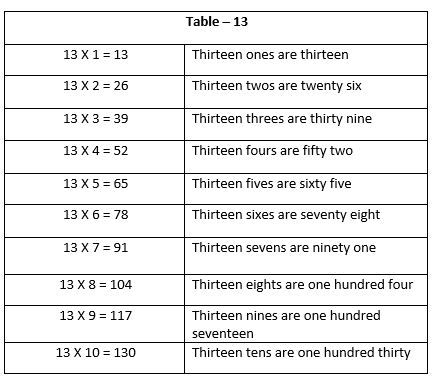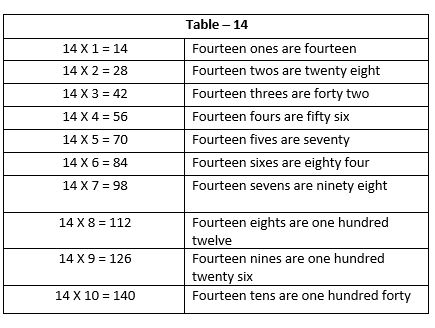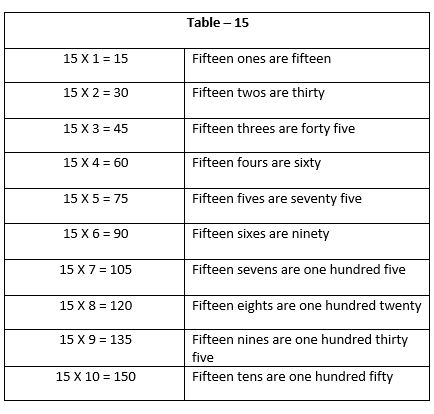## Multiplication of 2-Digit Number by 1-Digit Number

Follow the below mentioned rules for this kind of multiplication where there is no carry over.

1. Write both the numbers according to the place value.
2. Bigger number should occupy the upper row and single digit number should occupy the second row as shown below

3. Multiply the single digit present in 2nd row with the ones place of the 2-digit number. Write the result in ones place

4. Multiply the single digit present in 2nd row with the tens place of the 2-digit number. Write the result in tens place

Example 1. Multiply 34 by 2.

Solution.
1. Write 34 and 2 according to their place value. 34 should remain in the top row.
2. Multiply 2 with 4 = 2 X 4 = 8.Write 8 in the ones column.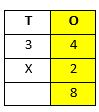3. Multiply 2 with 3 = 2 X 3 = 6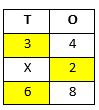Write 6 in tens place as shown.

Example 2.Multiply 26 by 3.

Solution.
1. Write 26 and 3 according to their place value. 26 should remain in the top row.

2. Multiply 3 by 6 = 3 X 6 = 18 = 10 ones + 8 Ones = 1 Ten + 8 Ones

Write 8 ones in ones place and 1 ten in tens place. This 1 ten is known as carry over.3. Multiply 3 by 2 = 3 X 2 = 7

Add 1 (carry over) to 7 = 1 + 7 = 8

Write 8 in tens column.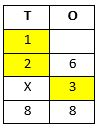## Multiplication of 3-Digit Number by 1-Digit Number

Process of multiplying 3-digit number by 1-digit number is like the process of multiplying 2-digit number by 1-digit number.
Let see some examples.

Example 1. Multiply 158 by 4.

Solution.
Step 1. Multiply ones column

8 X 4 = 32 = 3 tens + 2 ones

Write 2 in ones place and carry over 3 to tens place.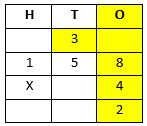Step 2. Multiply tens column

5 X 4 = 20

Add 3 tens (Carry over) with 20 tens = 20 + 3 = 23 tens = 20 tens + 3 tens

= 2 hundred + 3 tens

Write 3 tens in tens column and carry over 2 hundreds to hundred column.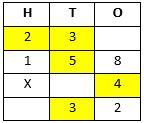Step 3. Multiply hundreds column. 1 x 4 = 4

Add 2 hundreds (Carry over) with 4 hundred

= 4 + 2 = 6 hundred

Write 6 hundred in hundreds column.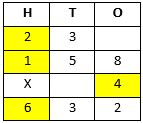So , the answer is 632.

## Multiplication by 10,20,30

When we multiply any number with 10,20,30, etc. then multiply the number with the multiplicand without zero and add zero at the end of result.

Example 1. Multiply 36 by 10.

Solution. 36 X 1 = 36

Now add one zero to the extreme right of 36 i.e. 360.

Example 2. Multiply 36 by 20.

Solution. 36 X 2 = 72

Add one zero to the extreme right of 72 i.e. 720.

Example 3. Multiply 36 by 30.

Solution. 36 X 3 = 108
Add one zero to the extreme right of 108 i.e. 1080.

## Word Problem

In our day to day life multiplication is used to solve different problems. Let’s have a look at some examples.

Example 1. In a color box there are 12 colors present. How many colors will be there in 5 such color boxes.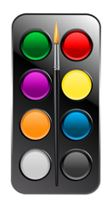Solution. Number of colors present in 1 box = 12

Number of colors present in 5 such boxes = No. of colors in 1 box X No. of boxes

= 12 X 5 = 60

So, 60 colors will be there in 5 color boxes.

Example 2. A jar contains 65 chocolates. How many chocolates do 8 similar jars contain?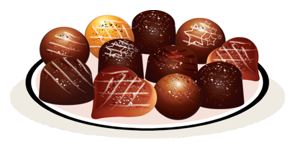Solution. No. of chocolates in a jar = 65

No. of chocolates present in 8 jars = No. of chocolates in a jar X No. of jars

= 65 X 8 = 520

So, there are 520 chocolates in 8 jars.

## Multiplication Test

Multiplication Test - 1

Multiplication Test - 2

## Class-2 Multiplication Worksheet

Multiplication Worksheet - 1

Multiplication Worksheet - 2

Multiplication Worksheet - 3